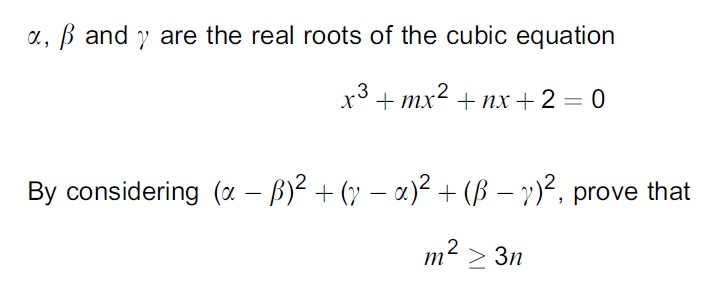# Practice Questions – Polynomials

1. The cubic polynomial  $f\left(x\right)$ is defined by  $f\left(x\right)={x}^{3}+a{x}^{2}+14x+a+1$
where $a$ is a constant. It is given that  $\left(x+2\right)$ is a factor of $f\left(x\right)$ .
(i) Use the factor theorem to find the value of a and hence factorise  $f\left(x\right)$ completely.[5 marks]

(ii) Hence, without using a calculator, solve the equation $f\left(2x\right)=3f\left(x\right)$ . [4 marks]

2.